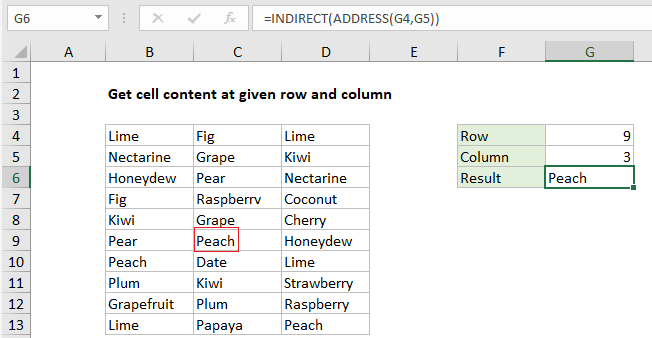## Excel Office

Excel How Tos, Tutorials, Tips & Tricks, Shortcuts

# Get cell content at given row and column in Excel

This tutorial shows how to Get cell content at given row and column in Excel using the example below;

## Formula

`=INDIRECT(ADDRESS(row,col))`## Explanation

To get cell content with a given row and column number, you can use the ADDRESS function together with INDIRECT. In the example shown, the formula in G6 is:

`=INDIRECT(ADDRESS(G4,G5))`

### How this formula works

The Excel ADDRESS function returns the address for a cell based on a given row and column number. For example, the ADDRESS function with 1 for both row and column like this:

`=ADDRESS(1,1)`

returns “\$A\$1” as text.

Also See:   Last row number in range

The INDIRECT function returns a valid reference from a text string.

In the example shown, the ADDRESS function returns the value “\$C\$9” inside INDIRECT:

`=INDIRECT("\$C\$9")`

The INDIRECT then this text into a normal reference and returns the value in cell C9, which is “Peach”.

Note: INDIRECT is a volatile function and can cause performance problems in more complicated worksheets.

Also See:   How to create dynamic reference table name in Excel

### With INDEX

By feeding the INDEX function an array that begins at A1, and includes cells to reference, you can get the same result with a formula that may be easier to understand. For example, the formula below will return the same result as seen in the screenshot.

`=INDEX(A1:E100,G4,G5)`

The size of the array is arbitrary, but it must to start at A1 and include the data you wish to reference.

Also See:   Find Closest Match in Excel Using INDEX, MATCH, ABS and MIN functions